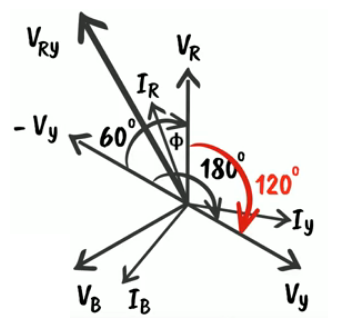Checkout JEE MAINS 2022 Question Paper Analysis : Checkout JEE MAINS 2022 Question Paper Analysis :

# Relation Between Line Voltage And Phase Voltage

For any polyphase system, Line voltage is defined as the voltage between two given phase. Whereas the phase voltage is the voltage between the given phase and neutral. The relation between line voltage and phase voltage are proportionate.

## Line Voltage and Phase Voltage

For a three phase or start connection, the Line voltage and Phase voltage relation can be mathematically written as-

 $$\begin{array}{l}V_{l}=\sqrt{3} V_{ph}\end{array}$$

Where,

• Vl is the Line voltage.
• Vph is the Phase voltage.
• Measure using the unit of voltage Volt (V).

### Line voltage and Phase voltage Relation

Line voltage and Phase voltage are directly proportional to each other. That means-

• When the line voltage increases, the phase voltage will also increase.
• When phase voltage increases, it will be reflected in the increase in line voltage.

## Line voltage and Phase voltage in a Star connection

Three phase voltage or star connection generally consists of voltage flowing through three different channels, for our simplicity we name it Voltage in the red line (VR), Voltage in Yellow line(VY), the voltage in blue line(VB). The voltage in all three channels is equal. Mathematically given as-

$$\begin{array}{l}V_{ph}=V_{R}=V_{Y}=V_{B}\end{array}$$Here, line current = Phase current

$$\begin{array}{l}\Rightarrow I_{R}=I_{Y}=I_{B}=I_{l}\end{array}$$

If we extend VY backward we get -VY and draw a resultant voltage of VR and VY we get-

$$\begin{array}{l}V_{RY}=V_{R}+(-V_{Y})\end{array}$$
$$\begin{array}{l}\Rightarrow V_{l}=\left | V_{RY} \right |=\sqrt{V_{R}^{2}+V_{Y}^{2}+2V_{R}V_{Y}Cos60}\end{array}$$
$$\begin{array}{l}=\sqrt{V_{ph}^{2}+V_{ph}^{2}+2V_{ph}V_{ph}\frac{1}{2}}\end{array}$$

Because

$$\begin{array}{l}cos 60=\frac{1}{2}\end{array}$$

Thus, we arrive at the relation between line voltage and phase voltage in star connection in an electric circuit

$$\begin{array}{l}V_{l}=\sqrt{3} V_{ph}\end{array}$$

Hope you understood the relation between line voltage and phase voltage in a polyphase system or connection.

Physics Related Topics:

Stay tuned with BYJU’S for more such interesting articles. Also, register to “BYJU’S-The Learning App” for loads of interactive, engaging physics related videos and an unlimited academic assist.

## Frequently Asked Questions – FAQs

### What is line voltage?

Line voltage is defined as the voltage between two given phase.

### What is phase voltage?

Phase voltage is the voltage between the given phase and neutral.

### When the line voltage increases, what happens to the phase voltage.

When the line voltage increases, the phase voltage will also increase.

### Give the relationship between the line voltage and phase voltage for a three phase or start connection?

$$\begin{array}{l}V_{l}=\sqrt{3} V_{ph}\end{array}$$

### State true or false: When phase voltage increases, it will be reflected in the increase in line voltage.

True.
Test Your Knowledge On Relation Between Line Voltage And Phase Voltage!